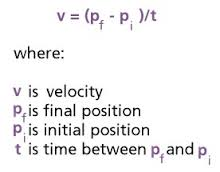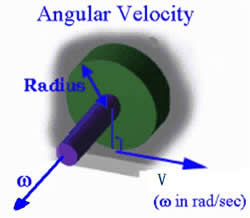# Difference between Velocity and Angular Velocity

##### Key Difference: Velocity refers to the rate of change of distance with respect to time. It is a vector quantity, which means that it has both - a direction and a magnitude. Angular velocity evaluates the rate of change of angular position for a spinning object with respect to time.Velocity refers to the rate of change of distance with respect to time. It is a vector quantity, which means that it has both - a direction and a magnitude. Velocity can also be simply described as the change in distance per unit time. Velocity keeps the track of direction, and thus direction plays a vital role in the calculation of the velocity. One needs to specify the direction in order to provide the complete information regarding the velocity of an object. The units of velocity are m/sec, cm/sec, ft/sec, km/h, miles/hr, etc.Angular velocity is also known as the rotational velocity. It evaluates the rate of change of angular position for a spinning object with respect to time. Angular velocity is related to the turning of an object, therefore it can be expressed in degrees or radians per unit time. Symbol for the Angular velocity is a lower case Greek symbol known as omega. Right-hand rule is used to describe the direction of rotation.

Comparison between Velocity and Angular Velocity:

 Velocity Angular Velocity Definition Velocity refers to the rate of change of distance with respect to time. It is a vector quantity, which means that it has both - a direction and a magnitude. Angular velocity evaluates the rate of change of angular position for a spinning object with respect to time. Formula Velocity = displacement/time Angular Velocity = Angular displacement/time Units m/sec, cm/sec, ft/sec, km/h, miles/hr, etc. S.I. unit is radians per second. Example If a car comes back to its initial position, then the displacement is zero. Therefore, this motion results in zero velocity. The angular velocity of the Earth’s rotation is  (2 X pi)/24 radians per hour. Direction Directed in the same direction in which an object is moving. Directed along the axis of rotation in a direction defined by the right hand screw rule.

Image Courtesy: btc.montana.edu, webspace.utexas.edu